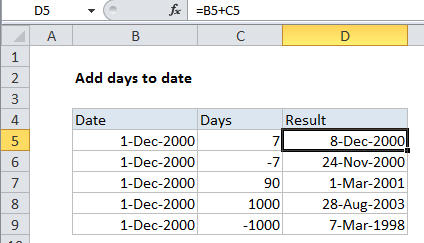## Excel Office

Excel How Tos, Tutorials, Tips & Tricks, Shortcuts

# Add days to date in Excel

It you need to add days to date in Excel then this tutorials is for you. See example below:

To add a given number of years to a date, you can use the EDATE function.

## Formula

`=date+days`## Explanation

In the example shown, the formula in D5 is:

`=B5+C5`

### How this formula works

Dates in Excel are just serial numbers. The number 1 represents January 1, 1900, the number 1000 is September 26, 1902, and so on.

Also See:   EDATE function: Description, Usage, Syntax, Examples and Explanation

When you have a valid date in Excel, you and just add days directly. Day values can be positive or negative.

For example, with a date in A1, you can add 100 days like so:

`=A1+100`

In the example shown, the formula is solved like this:

```=B5+C5
=36861+7
=36868```

Recall, Excel saves dates as number so when formatted as a date, 36868 is December 8, 2000.

Also See:   How to get same date next month or previous month in Excel